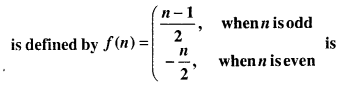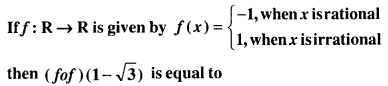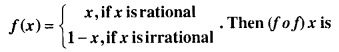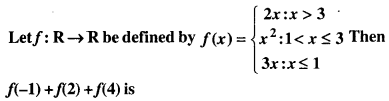# Bihar Board 12th Maths Objective Answers Chapter 1 Relations and Functions

Bihar Board 12th Maths Objective Questions and Answers

## Bihar Board 12th Maths Objective Answers Chapter 1 Relations and Functions

Question 1.
If R = {(x, y) : x, y ∈ I, x2 + y2 ≤ 4} is a relation in I, then domain of R is
(a) {0, 1, 2}
(b) {-2, -1, 0}
(c) {-2, -1, 0, 2}
(d) None of these
(c) {-2, -1, 0, 2}

Question 2.
If A = {1, 2, 3}, B = {1, 4, 6, 9} and R is relation from A to B defined by ‘or is greater thany’. The range of R is
(a) {1, 4, 6, 9}
(b) {4, 6, 9}
(c) {1}
(d) None of these
(c) {1}Question 3.
The relation R = {(1, 1), (2, 2), (3, 3), (1, 2), (2, 3), (1, 3)} on set A = {1, 2, 3} is
(a) Reflexive but not symmetric
(b) Reflexive but not transitive
(c) Symmetric and transitive
(d) Neither symmetric nor transitive
(a) Reflexive but not symmetric

Use this handy Domain and Range Calculator to get the exact answer for your function instantly.

Question 4.
Let P = {(x, y) | x2 + y2 = 1, xy ∈ R}. Then P is
(a) Reflexive
(b) Symmetric
(c) Transitive
(d) Anti-symmetric
(b) Symmetric

Question 5.
Let S be the set of all real numbers. Then, the relation R = {(a, b) : 1 + ab > 0} on S is
(a) Reflexive and symmetric but not transitive
(b) Reflexive and transitive but not symmetric
(c) Symmetric and transitive but not reflexive
(d) Reflexive, transitive and symmetric
(a) Reflexive and symmetric but not transitive

Question 6.
Let R be a relation on the set N be defined by {(x, y) | x, y ∈ N, 2x + y = 41}. Then, R is
(a) Reflexive
(b) Symmetric
(c) Transitive
(d) None of these
(d) None of theseQuestion 7.
Let R be the relation on the set of all real numbers defined by aRb iff |a – b| ≤ 1. Then, R is
(a) Reflexive and symmetric
(b) Symmetric only
(c) Transitive only
(d) Anti-symmetric only
(a) Reflexive and symmetric

Question 8.
If R and R’ are symmetric relations (not disjoint) on a set A, then the relation R ∩ R’ is
(a) Reflexive
(b) Symmetric
(c) Transitive
(d) None of these
(b) Symmetric

Question 9.
Let A = {1, 2, 3, 4} and R be a relation in A given by R = {(1, 1), (2, 2), (3, 3), (4, 4), (1, 2), (2, 1), (3, 1). Then, R is
(a) Reflexive
(b) Transitive
(c) An equivalence relation
(d) None of these
(a) ReflexiveQuestion 10.
Which one of the following relations on R is an equivalence relation?
(a) aR1b ⇔ |a| = |b|
(b) aR2b ⇔ a ≥ b
(c) aR3b ⇔ a divides b
(d) aR4b ⇔ a < b
(a) aR1b ⇔ |a| = |b|

The factors of 84 are 1, 2, 3, 4, 6, 7, 12, 14, 21, 28, 42, 84.

Question 11.
Let R be a relation on the set N of natural numbers denoted by nRm ⇔ n is a factor of m (i.e. n | m). Then, R is
(a) Reflexive and symmetric
(b) Transitive and symmetric
(c) Equivalence
(d) Reflexive, transitive but not symmetric
(d) Reflexive, transitive but not symmetric

Question 12.
Let S = {1, 2, 3, 4, 5} and let A = S × S. Define the relation R on A as follows:
(a, b) R (c, d) iff ad = cb. Then, R is
(a) reflexive only
(b) Symmetric only
(c) Transitive only
(d) Equivalence relation
(d) Equivalence relation

Question 13.
Let R be the relation “is congruent to” on the set of all triangles in a plane is
(a) reflexive
(b) symmetric
(c) symmetric and reflexive
(d) equivalence
(d) equivalenceQuestion 14.
Total number of equivalence relations defined in the set S = {a, b, c} is
(a) 5
(b) 3!
(c) 23
(d) 33
(a) 5

Question 15.
The relation R is defined on the set of natural numbers as {(a, b) : a = 2b}. Then, R-1 is given by
(a) {(2, 1), (4, 2), (6, 3),….}
(b) {(1, 2), (2, 4), (3, 6), ……..}
(c) R-1 is not defiend
(d) None of these
(b) {(1, 2), (2, 4), (3, 6), ……..}

Question 16.
The domain of the function $$f(x)=\frac{1}{\sqrt{\{\sin x\}+\{\sin (\pi+x)\}}}$$ where {.} denotes fractional part, is
(a) [0, π]
(b) (2n + 1) π/2, n ∈ Z
(c) (0, π)
(d) None of these
(d) None of these

Question 17.
Range of $$f(x)=\sqrt{(1-\cos x) \sqrt{(1-\cos x) \sqrt{(1-\cos x) \ldots \ldots \infty}}}$$
(a) [0, 1]
(b) (0, 1)
(c) [0, 2]
(d) (0, 2)
(c) [0, 2]

Question 18.
Consider the function y = f(x) satisfying the condition $$f\left(x+\frac{1}{x}\right)=x^{2}+\frac{1}{x^{2}}(x \neq 0)$$. Then which of the
following is not true?
(a) Domain of f(x) is (-∞, -2] ∪ [2, ∞)
(b) f(x) is an even function
(c) Range of f(x) is [2, ∞)
(d) None of these
(d) None of theseQuestion 19.
The function f : R → R defined by f(x) = 6x + 6 |x| is
(a) One-one and onto
(b) Many-one and onto
(c) One-one and into
(d) Many-one and into
(c) One-one and into

Question 20.
f : N → N where f(x) = x – (-1)x, then f is
(a) One-one and into
(b) Many-one and into
(c) One-one and onto
(d) Many-one and onto
(c) One-one and onto

Question 21.
A function f from the set of natural numbers to integers is defined by(a) one-one but not onto
(b) onto but not one-one
(c) one-one and onto both
(d) neither one-one nor onto
(c) one-one and onto both

Question 22.
Let X = {-1, 0, 1}, Y = {0, 2} and a function f : X → Y defiend by y = 2x4, is
(a) one-one onto
(b) one-one into
(c) many-one onto
(d) many-one into
(c) many-one onto

Question 23.
Let f : R → R be a function defined by $$f(x)=\frac{e^{|x|}-e^{-x}}{e^{x}+e^{-x}}$$ then f(x) is
(a) one-one onto
(b) one-one but not onto
(c) onto but not one-one
(d) None of these
(d) None of theseQuestion 24.
Let g(x) = x2 – 4x – 5, then
(a) g is one-one on R
(b) g is not one-one on R
(c) g is bijective on R
(d) None of these
(b) g is not one-one on R

Question 25.
Let A = R – {3}, B = R – {1}. Let f : A → B be defined by $$f(x)=\frac{x-2}{x-3}$$. Then,
(a) f is bijective
(b) f is one-one but not onto
(c) f is onto but not one-one
(d) None of these
(a) f is bijective

Question 26.
The mapping f : N → N is given by f(n) = 1 + n2, n ∈ N when N is the set of natural numbers is
(a) one-one and onto
(b) onto but not one-one
(c) one-one but not onto
(d) neither one-one nor onto
(c) one-one but not onto

Question 27.
The function f : R → R given by f(x) = x3 – 1 is
(a) a one-one function
(b) an onto function
(c) a bijection
(d) neither one-one nor onto
(c) a bijection

Question 28.
Let f : [0, ∞) → [0, 2] be defined by $$f(x)=\frac{2 x}{1+x}$$, then f is
(a) one-one but not onto
(b) onto but not one-one
(c) both one-one and onto
(d) neither one-one nor onto
(a) one-one but not onto

Question 29.
If N be the set of all-natural numbers, consider f : N → N such that f(x) = 2x, ∀ x ∈ N, then f is
(a) one-one onto
(b) one-one into
(c) many-one onto
(d) None of these
(b) one-one intoQuestion 30.
Let A = {x : -1 ≤ x ≤ 1} and f : A → A is a function defined by f(x) = x |x| then f is
(a) a bijection
(b) injection but not surjection
(c) surjection but not injection
(d) neither injection nor surjection
(a) a bijection

Question 31.
Let f : R → R be a function defined by f(x) = x3 + 4, then f is
(a) injective
(b) surjective
(c) bijective
(d) none of these
(c) bijective

Question 32.
The function f : A → B defined by f(x) = 4x + 7, x ∈ R is
(a) one-one
(b) Many-one
(c) Odd
(d) Even
(a) one-one

Question 33.
The smallest integer function f(x) = [x] is
(a) One-one
(b) Many-one
(c) Both (a) & (b)
(d) None of these
(b) Many-one

Question 34.
The function f : R → R defined by f(x) = 3 – 4x is
(a) Onto
(b) Not onto
(c) None one-one
(d) None of these
(a) OntoQuestion 35.
The number of bijective functions from set A to itself when A contains 106 elements is
(a) 106
(b) (106)2
(c) 106!
(d) 2106
(c) 106!

Question 36.
If f(x) = (ax2 + b)3, then the function g such that f(g(x)) = g(f(x)) is given by
(a) $$g(x)=\left(\frac{b-x^{1 / 3}}{a}\right)$$
(b) $$g(x)=\frac{1}{\left(a x^{2}+b\right)^{3}}$$
(c) $$g(x)=\left(a x^{2}+b\right)^{1 / 3}$$
(d) $$g(x)=\left(\frac{x^{1 / 3}-b}{a}\right)^{1 / 2}$$
(d) $$g(x)=\left(\frac{x^{1 / 3}-b}{a}\right)^{1 / 2}$$

Question 37.
If f : R → R, g : R → R and h : R → R is such that f(x) = x2, g(x) = tanx and h(x) = logx, then the value of [ho(gof)](x), if x = $$\frac{\sqrt{\pi}}{2}$$ will be
(a) 0
(b) 1
(c) -1
(d) 10
(a) 0

Question 38.
If f : R → R and g : R → R defined by f(x) = 2x + 3 and g(x) = x2 + 7, then the value of x for which f(g(x)) = 25 is
(a) ±1
(b) ±2
(c) ±3
(d) ±4
(b) ±2

Question 39.
Let the functions f, g, h are defined from R to R such thatho(fog)(x) is defined by
(a) x
(b) x2
(c) 0
(d) none of these
(b) x2Question 40.(a) 1
(b) -1
(c) √3
(d) 0
(b) -1

Question 41.
Let f : N → R : f(x) = $$\frac{(2 x-1)}{2}$$ and g : Q → R : g(x) = x + 2 be two functions. Then, (gof) ($$\frac{3}{2}$$) is
(a) 3
(b) 1
(c) $$\frac{7}{2}$$
(d) None of these
(a) 3

Question 42.
Let $$f(x)=\frac{x-1}{x+1}$$, then f(f(x)) is
(a) $$\frac{1}{x}$$
(b) $$-\frac{1}{x}$$
(c) $$\frac{1}{x+1}$$
(d) $$\frac{1}{x-1}$$
(b) $$-\frac{1}{x}$$

Question 43.
If f(x) = $$1-\frac{1}{x}$$, then f(f($$\frac{1}{x}$$))
(a) $$\frac{1}{x}$$
(b) $$\frac{1}{1+x}$$
(c) $$\frac{x}{x-1}$$
(d) $$\frac{1}{x-1}$$
(c) $$\frac{x}{x-1}$$

Question 44.
If f : R → R, g : R → R and h : R → R are such that f(x) = x2, g(x) = tan x and h(x) = log x, then the value of (go(foh)) (x), if x = 1 will be
(a) 0
(b) 1
(c) -1
(d) π
(a) 0Question 45.
If f(x) = $$\frac{3 x+2}{5 x-3}$$ then (fof)(x) is
(a) x
(b) -x
(c) f(x)
(d) -f(x)
(a) x

Question 46.
If f(x) = (ax2 – b)3, then the function g such that f{g(x)} = g{f(x)} is given by
(a) $$g(x)=\left(\frac{b-x^{1 / 3}}{a}\right)^{1 / 2}$$
(b) $$g(x)=\frac{1}{\left(a x^{2}+b\right)^{3}}$$
(c) $$g(x)=\left(a x^{2}+b\right)^{1 / 3}$$
(d) $$g(x)=\left(\frac{x^{1 / 3}+b}{a}\right)^{1 / 2}$$
(d) $$g(x)=\left(\frac{x^{1 / 3}+b}{a}\right)^{1 / 2}$$

Question 47.
If f : [1, ∞) → [2, ∞) is given by f(x) = x + $$\frac{1}{x}$$, then f-1 equals to
(a) $$\frac{x+\sqrt{x^{2}-4}}{2}$$
(b) $$\frac{x}{1+x^{2}}$$
(c) $$\frac{x-\sqrt{x^{2}-4}}{2}$$
(d) $$1+\sqrt{x^{2}-4}$$
(a) $$\frac{x+\sqrt{x^{2}-4}}{2}$$

Question 48.
Let f(x) = x2 – x + 1, x ≥ $$\frac{1}{2}$$, then the solution of the equation f(x) = f-1(x) is
(a) x = 1
(b) x = 2
(c) x = $$\frac{1}{2}$$
(d) None of these
(a) x = 1

Question 49.
Which one of the following function is not invertible?
(a) f : R → R, f(x) = 3x + 1
(b) f : R → [0, ∞), f(x) = x2
(c) f : R+ → R+, f(x) = $$\frac{1}{x^{3}}$$
(d) None of these
(d) None of these

Question 50.
The inverse of the function $$y=\frac{10^{x}-10^{-x}}{10^{x}+10^{-x}}$$ is
(a) $$\log _{10}(2-x)$$
(b) $$\frac{1}{2} \log _{10}\left(\frac{1+x}{1-x}\right)$$
(c) $$\frac{1}{2} \log _{10}(2 x-1)$$
(d) $$\frac{1}{4} \log \left(\frac{2 x}{2-x}\right)$$
(b) $$\frac{1}{2} \log _{10}\left(\frac{1+x}{1-x}\right)$$

Question 51.
If f : R → R defind by f(x) = $$\frac{2 x-7}{4}$$ is an invertible function, then find f-1.
(a) $$\frac{4 x+5}{2}$$
(b) $$\frac{4 x+7}{2}$$
(c) $$\frac{3 x+2}{2}$$
(d) $$\frac{9 x+3}{5}$$
(b) $$\frac{4 x+7}{2}$$Question 52.
Consider the function f in A = R – {$$\frac{2}{3}$$} defiend as $$f(x)=\frac{4 x+3}{6 x-4}$$. Find f-1.
(a) $$\frac{3+4 x}{6 x-4}$$
(b) $$\frac{6 x-4}{3+4 x}$$
(c) $$\frac{3-4 x}{6 x-4}$$
(d) $$\frac{9+2 x}{6 x-4}$$
(a) $$\frac{3+4 x}{6 x-4}$$

Question 53.
If f is an invertible function defined as f(x) = $$\frac{3 x-4}{5}$$, then f-1(x) is
(a) 5x + 3
(b) 5x + 4
(c) $$\frac{5 x+4}{3}$$
(d) $$\frac{3 x+2}{3}$$
(c) $$\frac{5 x+4}{3}$$

Question 54.
If f : R → R defined by f(x) = $$\frac{3 x+5}{2}$$ is an invertible function, then find f-1.
(a) $$\frac{2 x-5}{3}$$
(b) $$\frac{x-5}{3}$$
(c) $$\frac{5 x-2}{3}$$
(d) $$\frac{x-2}{3}$$
(a) $$\frac{2 x-5}{3}$$

Question 55.
Let f : R → R, g : R → R be two functions such that f(x) = 2x – 3, g(x) = x3 + 5. The function (fog)-1 (x) is equal to
(a) $$\left(\frac{x+7}{2}\right)^{1 / 3}$$
(b) $$\left(x-\frac{7}{2}\right)^{1 / 3}$$
(c) $$\left(\frac{x-2}{7}\right)^{1 / 3}$$
(d) $$\left(\frac{x-7}{2}\right)^{1 / 3}$$
(d) $$\left(\frac{x-7}{2}\right)^{1 / 3}$$

Question 56.
Let * be a binary operation on set of integers I, defined by a * b = a + b – 3, then find the value of 3 * 4.
(a) 2
(b) 4
(c) 7
(d) 6
(c) 7

Question 57.
If * is a binary operation on set of integers I defined by a * b = 3a + 4b – 2, then find the value of 4 * 5.
(a) 35
(b) 30
(c) 25
(d) 29
(b) 30Question 58.
Let * be the binary operation on N given by a * b = HCF (a, b) where, a, b ∈ N. Find the value of 22 * 4.
(a) 1
(b) 2
(c) 3
(d) 4
(b) 2

Question 59.
Consider the binary operation * on Q defind by a * b = a + 12b + ab for a, b ∈ Q. Find 2 * $$\frac{1}{3}$$
(a) $$\frac{20}{3}$$
(b) 4
(c) 18
(d) $$\frac{16}{3}$$
(a) $$\frac{20}{3}$$

Question 60.
Consider a binary operation * on the set {1, 2, 3, 4, 5} given by the following multiplication table. Compute (2 * 3) * (4 * 5).(a) 1
(b) 2
(c) 3
(d) 4
(a) 1

Question 61.
If the binary operation * is defind on the set Q+ of all positive rational numbers by a * b = $$\frac{a b}{4}$$. Then, $$3 *\left(\frac{1}{5} * \frac{1}{2}\right)$$ is equal to
(a) $$\frac{3}{160}$$
(b) $$\frac{5}{160}$$
(c) $$\frac{3}{10}$$
(d) $$\frac{3}{40}$$
(a) $$\frac{3}{160}$$

Question 62.
The number of binary operations that can be defined on a set of 2 elements is
(a) 8
(b) 4
(c) 16
(d) 64
(c) 16Question 63.
Let * be a binary operation on Q, defined by a * b = $$\frac{3 a b}{5}$$ is
(a) Commutative
(b) Associative
(c) Both (a) and (b)
(d) None of these
(c) Both (a) and (b)

Question 64.(a) 0
(b) 1
(c) 2
(d) 3
(a) 0

Question 65.
Let * be a binary operation on set Q of rational numbers defined as a * b = $$\frac{a b}{5}$$. Write the identity for *.
(a) 5
(b) 3
(c) 1
(d) 6
(a) 5

Question 66.
For binary operation * defind on R – {1} such that a * b = $$\frac{a}{b+1}$$ is
(a) not associative
(b) not commutative
(c) commutative
(d) both (a) and (b)
(d) both (a) and (b)Question 67.
The binary operation * defind on set R, given by a * b = $$\frac{a+b}{2}$$ for all a,b ∈ R is
(a) commutative
(b) associative
(c) Both (a) and (b)
(d) None of these
(a) commutative

Question 68.
Let A = N × N and * be the binary operation on A defined by (a, b) * (c, d) = (a + c, b + d). Then * is
(a) commutative
(b) associative
(c) Both (a) and (b)
(d) None of these
(c) Both (a) and (b)

Question 69.
Find the identity element in the set I+ of all positive integers defined by a * b = a + b for all a, b ∈ I+.
(a) 1
(b) 2
(c) 3
(d) 0
(d) 0

Question 70.
Let * be a binary operation on set Q – {1} defind by a * b = a + b – ab : a, b ∈ Q – {1}. Then * is
(a) Commutative
(b) Associative
(c) Both (a) and (b)
(d) None of these
(c) Both (a) and (b)Question 71.
The binary operation * defined on N by a * b = a + b + ab for all a, b ∈ N is
(a) commutative only
(b) associative only
(c) both commutative and associative
(d) none of these
(c) both commutative and associative

Question 72.
The number of commutative binary operation that can be defined on a set of 2 elements is
(a) 8
(b) 6
(c) 4
(d) 2
(d) 2

Question 73.
Let T be the set of all triangles in the Euclidean plane, and let a relation R on T be defined as aRb if a is congruent to b ∀ a, b ∈ T. Then R is
(a) reflexive but not transitive
(b) transitive but not symmetric
(c) equivalence
(d) None of these
(c) equivalence

Question 74.
The maximum number of equivalence relations on the set A = {1, 2, 3} are
(a) 1
(b) 2
(c) 3
(d) 5
(d) 5

Question 75.
Let us define a relation R in R as aRb if a ≥ b. Then R is
(a) an equivalence relation
(b) reflexive, transitive but not symmetric
(c) symmetric, transitive but not reflexive
(d) neither transitive nor reflexive but symmetric
(b) reflexive, transitive but not symmetricQuestion 76.
Let A = {1, 2, 3} and consider the relation R = {(1, 1), (2, 2), (3, 3), (1, 2), (2, 3), (1, 3)}. Then R is
(a) reflexive but not symmetric
(b) reflexive but not transitive
(c) symmetric and transitive
(d) neither symmetric, nor transitive
(a) reflexive but not symmetric

Question 77.
The identity element for the binary operation * defined on Q – {0} as a * b = $$\frac{a b}{2}$$ ∀ a, b ∈ Q – {0) is
(a) 1
(b) 0
(c) 2
(d) None of these
(c) 2

Question 78.
Let A = {1, 2, 3, …. n} and B = {a, b}. Then the number of surjections from A into B is
(a) $$^{n} P_{2}$$
(b) 2n – 2
(c) 2n – 1
(d) none of these
(b) 2n – 2Question 79.
Let f : R → R be defind by f(x) = $$\frac{1}{x}$$ ∀ x ∈ R. Then f is
(a) one-one
(b) onto
(c) bijective
(d) f is not defined
(d) f is not defined

Question 80.
Which of the following functions from Z into Z are bijective?
(a) f(x) = x3
(b) f(x) = x + 2
(c) f(x) = 2x + 1
(d) f(x) = x2 + 1
(b) f(x) = x + 2

Question 81.
Let f : R → R be the functions defined by f(x) = x3 + 5. Then f-1(x) is
(a) $$(x+5)^{\frac{1}{3}}$$
(b) $$(x-5)^{\frac{1}{3}}$$
(c) $$(5-x)^{\frac{1}{3}}$$
(d) 5 – x
(b) $$(x-5)^{\frac{1}{3}}$$

Question 82.
Let f : R – {$$\frac{3}{5}$$} → R be defined by f(x) = $$\frac{3 x+2}{5 x-3}$$. Then
(a) f-1(x) = f(x)
(b) f-1(x) = -f(x)
(c) (fof) x = -x
(d) f-1(x) = $$\frac{1}{19}$$ f(x)
(a) f-1(x) = f(x)Question 83.
Let f : [0, 1] → [0, 1] be defined by(a) constant
(b) 1 + x
(c) x
(d) None of these
(c) x

Question 84.(a) 9
(b) 14
(c) 5
(d) None of these
(a) 9Question 85.
Let f : R → R be given by f(x) = tan x. Then f-1(1) is
(a) $$\frac{\pi}{4}$$
(b) {nπ + $$\frac{\pi}{4}$$; n ∈ Z}
(c) Does not exist
(d) None of these
(b) {nπ + $$\frac{\pi}{4}$$; n ∈ Z}